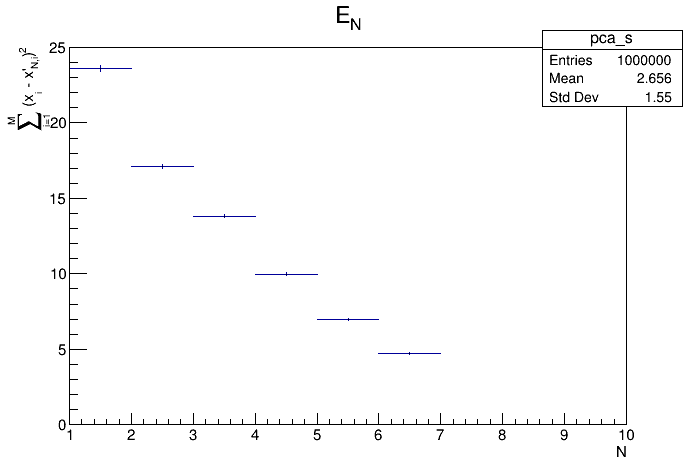# principal¶

Principal Components Analysis (PCA) example

Example of using TPrincipal as a stand alone class.

We create n-dimensional data points, where c = trunc(n / 5) + 1 are correlated with the rest n - c randomly distributed variables.

Author: Rene Brun, Christian Holm Christensen
This notebook tutorial was automatically generated with ROOTBOOK-izer from the macro found in the ROOT repository on Wednesday, August 17, 2022 at 09:34 AM.

Arguments are defined.

In :
Int_t n=10;
Int_t m=10000;

In :
Int_t c = n / 5 + 1;

cout << "*************************************************" << endl;
cout << "*         Principal Component Analysis          *" << endl;
cout << "*                                               *" << endl;
cout << "*  Number of variables:           " << setw(4) << n
<< "          *" << endl;
cout << "*  Number of data points:         " << setw(8) << m
<< "      *" << endl;
cout << "*  Number of dependent variables: " << setw(4) << c
<< "          *" << endl;
cout << "*                                               *" << endl;
cout << "*************************************************" << endl;

*************************************************
*         Principal Component Analysis          *
*                                               *
*  Number of variables:             10          *
*  Number of data points:            10000      *
*  Number of dependent variables:    3          *
*                                               *
*************************************************


Initilase the TPrincipal object. Use the empty string for the final argument, if you don't wan't the covariance matrix. Normalising the covariance matrix is a good idea if your variables have different orders of magnitude.

In :
TPrincipal* principal = new TPrincipal(n,"ND");


Use a pseudo-random number generator

In :
TRandom* randumNum = new TRandom;


Make the m data-points Make a variable to hold our data Allocate memory for the data point

In :
Double_t* data = new Double_t[n];
for (Int_t i = 0; i < m; i++) {

// First we create the un-correlated, random variables, according
// to one of three distributions
for (Int_t j = 0; j < n - c; j++) {
if (j % 3 == 0)      data[j] = randumNum->Gaus(5,1);
else if (j % 3 == 1) data[j] = randumNum->Poisson(8);
else                 data[j] = randumNum->Exp(2);
}

// Then we create the correlated variables
for (Int_t j = 0 ; j < c; j++) {
data[n - c + j] = 0;
for (Int_t k = 0; k < n - c - j; k++) data[n - c + j] += data[k];
}

}

input_line_55:2:2: warning: 'data' shadows a declaration with the same name in the 'std' namespace; use '::data' to reference this declaration
Double_t* data = new Double_t[n];
^


We delete the data after use, since TPrincipal got it by now.

In :
delete [] data;

input_line_56:2:12: error: reference to 'data' is ambiguous
delete [] data;
^
input_line_55:2:12: note: candidate found by name lookup is '__cling_N525::data'
Double_t* data = new Double_t[n];
^
/usr/include/c++/9/bits/range_access.h:318:5: note: candidate found by name lookup is 'std::data'
data(initializer_list<_Tp> __il) noexcept
^
/usr/include/c++/9/bits/range_access.h:289:5: note: candidate found by name lookup is 'std::data'
data(_Container& __cont) noexcept(noexcept(__cont.data()))
^
/usr/include/c++/9/bits/range_access.h:299:5: note: candidate found by name lookup is 'std::data'
data(const _Container& __cont) noexcept(noexcept(__cont.data()))
^
/usr/include/c++/9/bits/range_access.h:309:5: note: candidate found by name lookup is 'std::data'
data(_Tp (&__array)[_Nm]) noexcept
^


Do the actual analysis

In :
principal->MakePrincipals();


Print out the result on

In :
principal->Print();

 Variable #  | Mean Value |   Sigma    | Eigenvalue
-------------+------------+------------+------------
0 |      5.008 |      1.005 |     0.3851
1 |      7.998 |      2.861 |     0.1107
2 |      1.967 |      1.956 |     0.1036
3 |      5.016 |      1.005 |     0.1015
4 |      8.009 |      2.839 |     0.1008
5 |      2.013 |      1.973 |    0.09962
6 |      4.992 |      1.014 |    0.09864
7 |         35 |      5.156 |  6.481e-16
8 |      30.01 |      5.049 |  2.202e-16
9 |         28 |      4.649 |  5.497e-16



Test the PCA

In :
principal->Test();

Info in <TCanvas::MakeDefCanvas>:  created default TCanvas with name c1


Make some histograms of the orginal, principal, residue, etc data

In :
principal->MakeHistograms();


Make two functions to map between feature and pattern space

In :
principal->MakeCode();

Writing on file "pca.C" ... done


Start a browser, so that we may browse the histograms generated above

In :
TBrowser* b = new TBrowser("principalBrowser", principal);

Warning in <TBrowser::TBrowser>: The ROOT browser cannot run in batch mode


Draw all canvases

In :
gROOT->GetListOfCanvases()->Draw()